Open in App
Not now

# Class 9 RD Sharma Solutions – Chapter 17 Constructions- Exercise 17.2

• Last Updated : 26 May, 2021

### Question 1. Draw an angle and label it as ∠BAC. Construct another angle, equal to ∠BAC

Solution: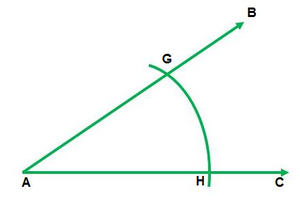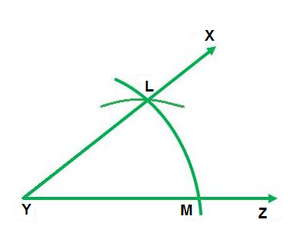Steps of construction:

1. Draw any ∠BAC and a line segment YZ .

2. Now, from center A draw an arc of any radius which intersects ∠BAC at points G and H.

3. Now, draw an arc of same radius with Y as a centre which intersects YZ at point M.

4. With M as center and radius equal to HG, draw an arc which intersects the previous arc at point L.

5. Draw a line segment joining points Y and L.

Hence ∠XYZ = ∠BAC

### Question 2. Draw an obtuse angle. Bisect it. Measure each of the angles so formed.

Solution: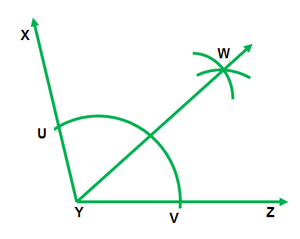Steps of construction:

1. Draw an angle ∠XYZ of 120°.

2. With Y as a centre and any radius, draw an arc which intersects XY at point U and YZ at point V.

3. With U as center and radius more than half of UV draw an arc.

4. With V as a center and same radius draw an arc which cuts the previous arc at point W.

5. Join YW.

Hence ∠XYW = ∠WYZ = 60°

### Question 3. Using your protractor, draw an angle of 108°. With this given angle as given, draw an angle of 54°.

Solution: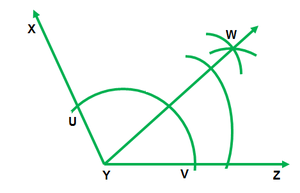Steps of construction:

1. Draw an ∠XYZ of 108°.

2. With Y as the center and any radius draw an arc which intersects XY at point U and YZ at point V.

3. With U as center and radius more than half of UV draw an arc.

4. With V as the centre and same radius draw an arc which intersects the previous arc at point W.

5. Join YW.

### Question 4. Using the protractor, draw a right angle. Bisect it to get an angle of measure 45°.

Solution: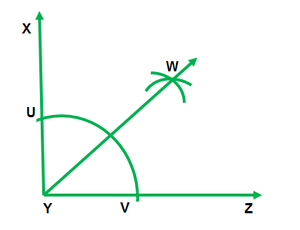Steps of construction:

1. Draw an ∠XYZ of 90°.

2. With Y as the centre and any radius draw an arc which intersects XY at point U and YZ at point V.

3. With U as center and radius more than half of UV draw an arc.

4. With V as center and same radius draw an arc which intersects the previous arc at point W.

5. Join YW.

Hence, ∠WYZ = 45°

### Question 5. Draw a linear pair of angles. Bisect each of the two angles. Verify that the two bisecting rays are perpendicular to each other.

Solution: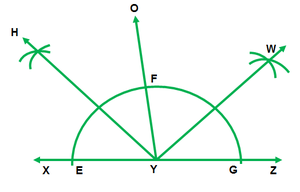Steps of construction:

1. Draw two ∠OYX and ∠OYZ forming linear pair

2. With center Y and any radius draw an arc which intersects XY at E and YO at point F and YZ at point G

3. With center E and F and any radius draw two arcs which intersect each other at point H

4. Join HY

5. With F and G as center and any radius draw two arcs which intersect each other at point W

6. Join WY

Hence, ∠HYW = 90°.

### Question 6. Draw a pair of vertically opposite angles. Bisect each of the two angles. Verify that the bisecting rays are in the same line.

Solution: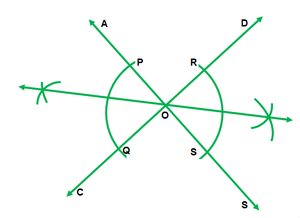Steps of Construction:

1. Draw a pair of vertically opposite angle ∠AOC and ∠DOB.

2. With O as the center and any radius draw two arcs which intersect OA at point P, OC at point Q, OB at point S and OD at point R.

3. With P and Q as center and radius more than half of PQ draw two arcs which intersect each other at point T.

4. Join TO.

5. With R and S as center and radius more than half of RS draw two arcs which intersect each other at point U.

6. Join OU.

Hence,TOU is a straight line

### Question 7. Using rulers and compasses only, draw a right angle.

Solution: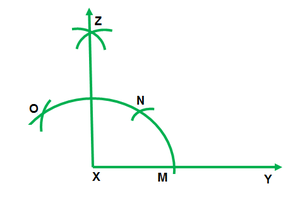Steps of construction:

1. Draw a line segment XY.

2. With X as the center and any radius draw an arc which intersects XY at point M.

3. With M as center and the same radius draw an arc which intersects the previous arc at point N.

4. With N as the center and the same radius draw an arc that intersects arc in (2) at point O.

5. With O and N as center and radius, more than half of ON draw arcs that intersect each other at point Z.

6. Join ZX.

Hence, ∠ZXM = 90°

### Question 8. Using rulers and compasses only, draw an angle of measure 135°.

Solution: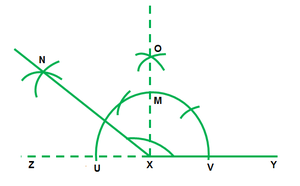Steps of construction:

1. Draw a line segment XY and produce YX to Z.

2. With X as the center and any radius draw an arc which intersects XZ at point U and XY at point V.

3. With U and V as center and radius more than half of UV draw arcs which intersect each other at point O.

4. Join OX which intersects the arc in step(2) at point M.

5. Keeping M and U as center and radius more than half of MU draw arcs which intersect each other at point N.

6. Join NX.

With ∠NXY = 135°

### Question 9. Using a protractor, draw an angle of measure 72°. With this angle as given draw angles of measure 36° and 54°.

Solution: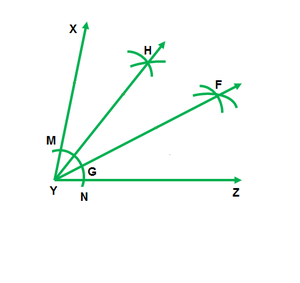Steps of construction:

1. Draw an ∠XYZ of 720 with the help of a protractor.

2. With Y as center and any radius draw an arc which intersects XY at point M and YZ at point N.

3. With M and N as center and radius more than half of MN draw two arcs which intersect each other at point F.

4. Join FY which intersects the arc in step(2) at point G.

5. With M and G as center and radius more than half of MG draw two arcs which intersect each other at point H.

6. Join HY

Hence, ∠HYX = 54° ∠FYZ = 36°

### (ii) 90°

Solution: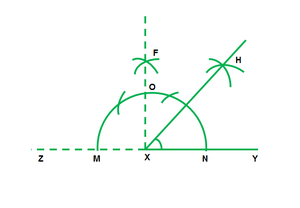(i) Steps of construction:

1. Draw a line segment XY and produce YX to point Z.

2. With X as the center and any radius draw an arc which intersects XZ at point M and XY at point N.

3. With M and N as center and radius more than half of MN draw arcs which intersect each other at point F.

4. Join FX which intersects the arc in step(2) at point O.

5. With O and N as center and radius more than half of ON draw arcs which intersect each other at point H.

6. Join HX.

Hence, ∠HXY = 45°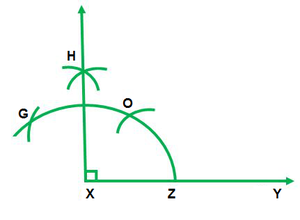(ii) Steps of construction

1. Draw a line segment XY.

2. With X as the center and any radius draw an arc which intersects XY at point Z.

3. With Z as center and the same radius draw an arc which intersects the previous arc at point O.

4. With O as the center and same radius draw an arc which intersects arc in (2) at point G.

5. With G and O as center and radius more than half of GO draw arcs which intersect each other at point H.

6. Join HX.

Hence, ∠HXY = 90°

### Question 11. Construct the angles of the following measurements:

(i) 30°

(ii) 75°

(iii) 105°

(iv) 135°

(v) 15°

(vi) 22(1/2)°

Solution: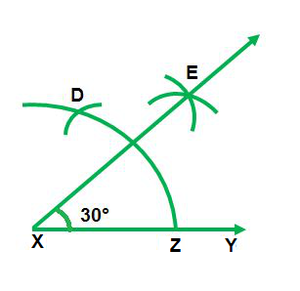(i) Steps of construction:

1. Draw a line segment XY.

2. With X as the centre and any radius draw an arc which intersects XY at point Z.

3. With Z as center and the same radius draw an arc which intersects the previous arc at point D.

4. With D and Z as center and radius more than half of DZ draw arcs which intersect each other at point E.

5. Join EX.

Hence, ∠EXY = 30°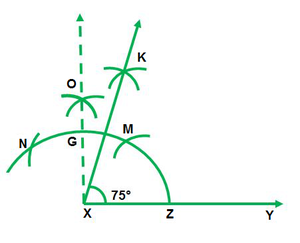(ii) Steps of construction:

1. Draw a line segment XY.

2. With x as center and any radius draw an arc which intersects XY at point Z.

3. With Z as center and the same radius draw an arc which intersects the previous arc at point M.

4. With M as center and same radius draw an arc which intersects arc in step (2) at point N.

5. With N and M as center and radius more than half of NM, draw arcs intersecting each other at point O.

6. Join OX which intersects arc in step(2) at point G.

7. With G and M as center and radius more than half of GM draw arcs intersecting each other at point K.

8. Join KX.

Hence, ∠KXY = 75°  .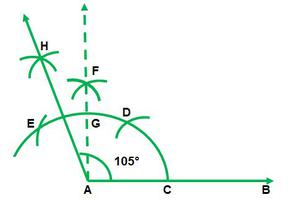(iii) Steps of construction:

1. Draw a line segment AB.

2. With A as the center and any radius draw an arc which intersects AB at point C.

3. With C as center and the same radius draw an arc which intersects the previous arc at point D.

4. With D as the centre and same radius draw an arc which intersects arc in step (2) at point E.

5. With E and D as center and radius more than half of ED draw arcs which intersect each other at point F.

6. Join FA which intersects arc in step (2) at point G.

7. With E and G as center and radius more than half of EG draw arcs which intersect each other at point H.

8. Join HA.

Hence, ∠HAB = 105°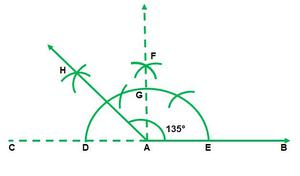(iv) Steps of construction:

1. Draw a line segment AB and produce BA to point C.

2. With A as the center and any radius draw an arc that intersects AC at D and AB at point E.

3. With D and E as center and radius more than half of DE draw arcs that intersect each other at point F.

4. Join FA which intersects the arc in step(2) at point G.

5. With G and D as center and radius more than half of GD draw arcs that intersect each other at point H

6. Join HA.

Hence, ∠HAB = 135°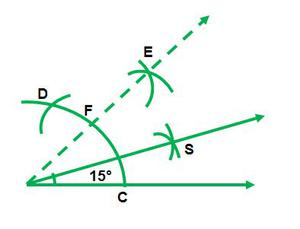(v) Steps of construction:

1. Draw a line segment AB.

2. With A as the centre and any radius draw an arc that intersects AB at point C.

3. With C as center and the same radius draw an arc that intersects the previous arc at point D.

4. With D and C as center and radius more than half of DC draw arcs that intersect each other at point E.

5. Join EA which intersects arc in step(2) at point F.

6. With F and C as center and radius more than half of FC draw arcs that intersect each other at point G.

7. Join GA.

Hence, ∠GAB = 15°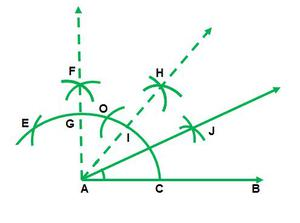(vi) Steps of construction:

1. Draw a line segment AB.

2. With A as the center and any radius draw an arc that intersects AB at point C.

3. With C as center and the same radius draw an arc that intersects the previous arc at point D.

4. With D as the centre and same radius draw an arc that intersects arc in step (2) at point E.

5. With E and D as center and radius more than half of ED draw arcs which intersect each at point F.

6. Join FA which intersects arc in step(2) at G.

7. With G and C as center and radius more than half of GC draw arcs intersecting each other at point H.

8. Join HA which intersects the arc in step(2) at a point I.

9. With I and C as center and radius more than half of IC draw arcs intersecting each other at point point J.

10. Join JA.

Hence, ∠JAB = 22(1/2)°

My Personal Notes arrow_drop_up